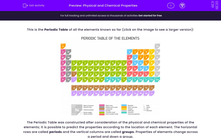# Use BIDMAS to Find the Order of Operations in Calculations

In this worksheet, students will use the order of BIDMAS to carry out mixed calculations.Key stage:  KS 2

Curriculum topic:   Number: Addition, Subtraction, Multiplication and Division

Curriculum subtopic:   Carry Out Calculations Using Order of Operations

Difficulty level:#### Worksheet Overview

The agreed order of working out more than one operation is as follows:

Brackets
Powers or Indices
Division and Multiplication

This can be remembered using the word: BIDMAS

However, remember that multiplication and division are equally important, so in a calculation just involving multiplication and division, carry this out from left to right.

The same is true of addition and subtraction, these two are also equally important, and so we again should carry this out from left to right.Examples

6 + 9 ÷ 3 = 6 + 3 = 9 (divide first)

(6 + 9) ÷ 3 = 15 ÷ 3 = 5 (brackets first)

15 - 4 × 2 = 15 - 8 = 7 (multiply first)

(15 - 4) × 2 = 11 × 2 = 22 (brackets first)

4 × 32 = 4 × 9 = 36 (power first)

(4 × 3)2 = 122 = 144 (brackets first)

14 - 4 + 5 = 15 (equally important, so left to right)

Does that make sense?Let's try some questions then!

### What is EdPlace?

We're your National Curriculum aligned online education content provider helping each child succeed in English, maths and science from year 1 to GCSE. With an EdPlace account you’ll be able to track and measure progress, helping each child achieve their best. We build confidence and attainment by personalising each child’s learning at a level that suits them.

Get started